# Age Estimation VGG-16 Trained onIMDB-WIKI and Looking at People Data

Predict a person's age from an image of their face

Originally released in 2015 as a pre-trained model for the launch of the IMDB-WIKI dataset by the Computer Vision Lab at ETH Zurich, this model is based on the VGG-16 architecture and is designed to run on cropped images of faces only. The model was then fine-tuned on the dataset for the 2015 Looking At People Age Estimation Challenge. An ensemble of these models won first place at the challenge.

Number of layers: 40 | Parameter count: 134,674,341 | Trained size: 540 MB |

## Training Set Information

• IMDB-WIKI, a dataset consisting of 520,000 images of faces, each featuring labels for gender and age. Looking at People Apparent Age V1, a dataset consisting of 5,000 images, each displaying a single individual, labeled with the apparent age.

## Examples

### Resource retrieval

Get the pre-trained net:

 In:=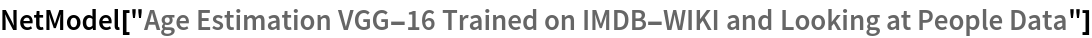Out=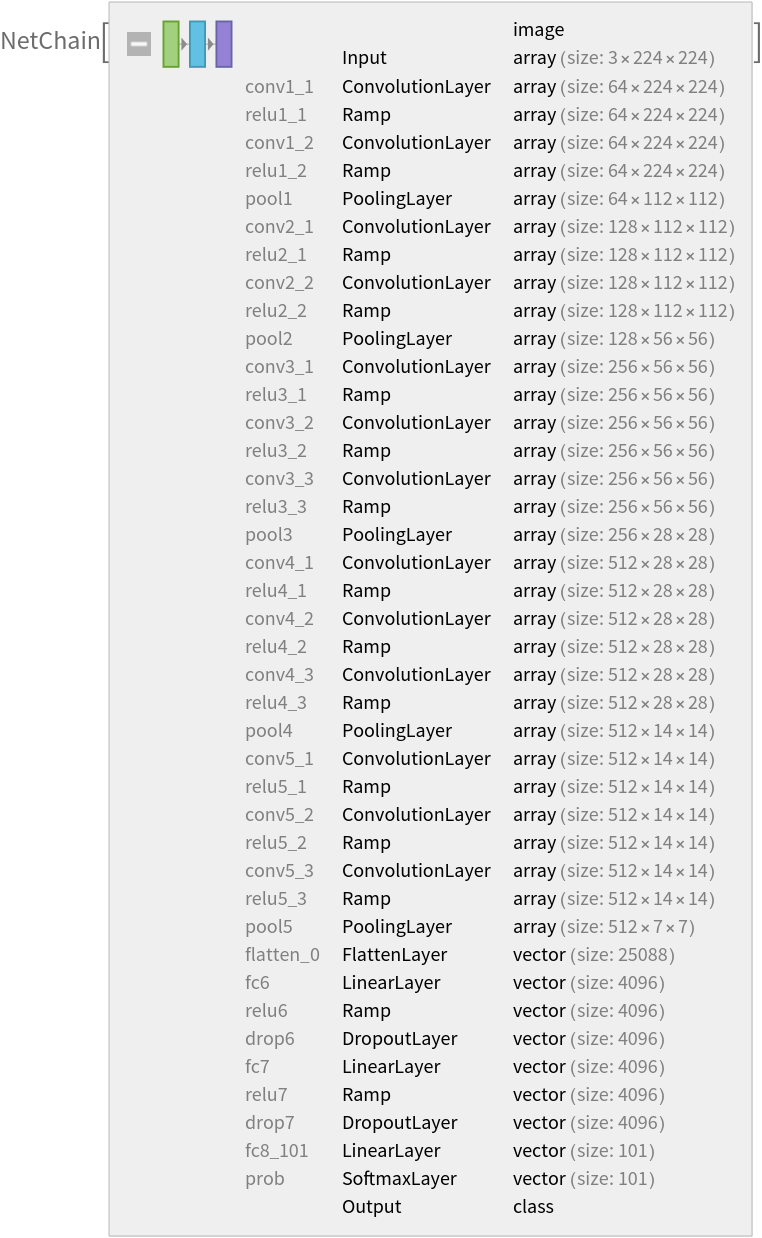### Basic usage

Guess the age of a person from a photograph. First, obtain the trained net:

 In:=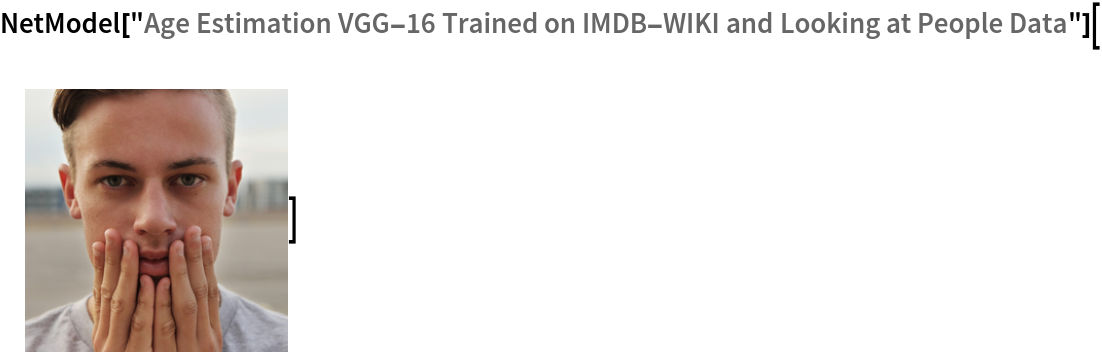Out=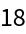Obtain the probability distribution over all possible ages:

 In:=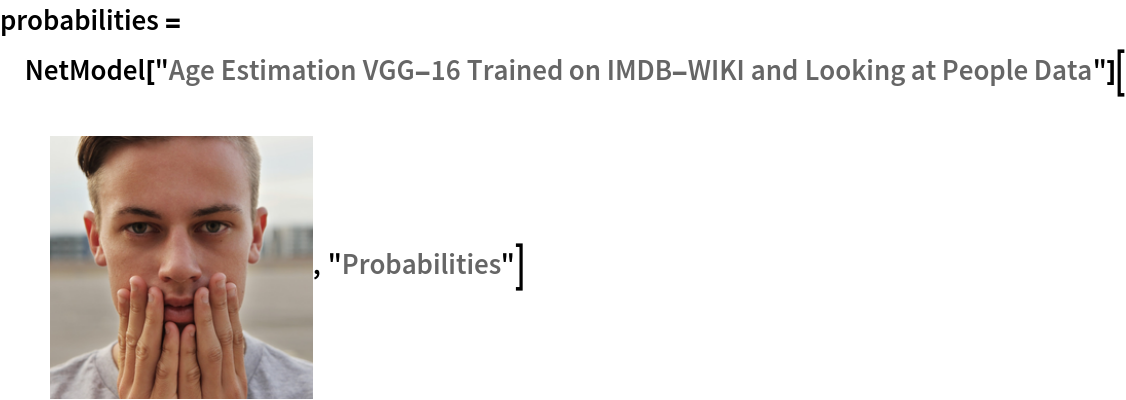Out=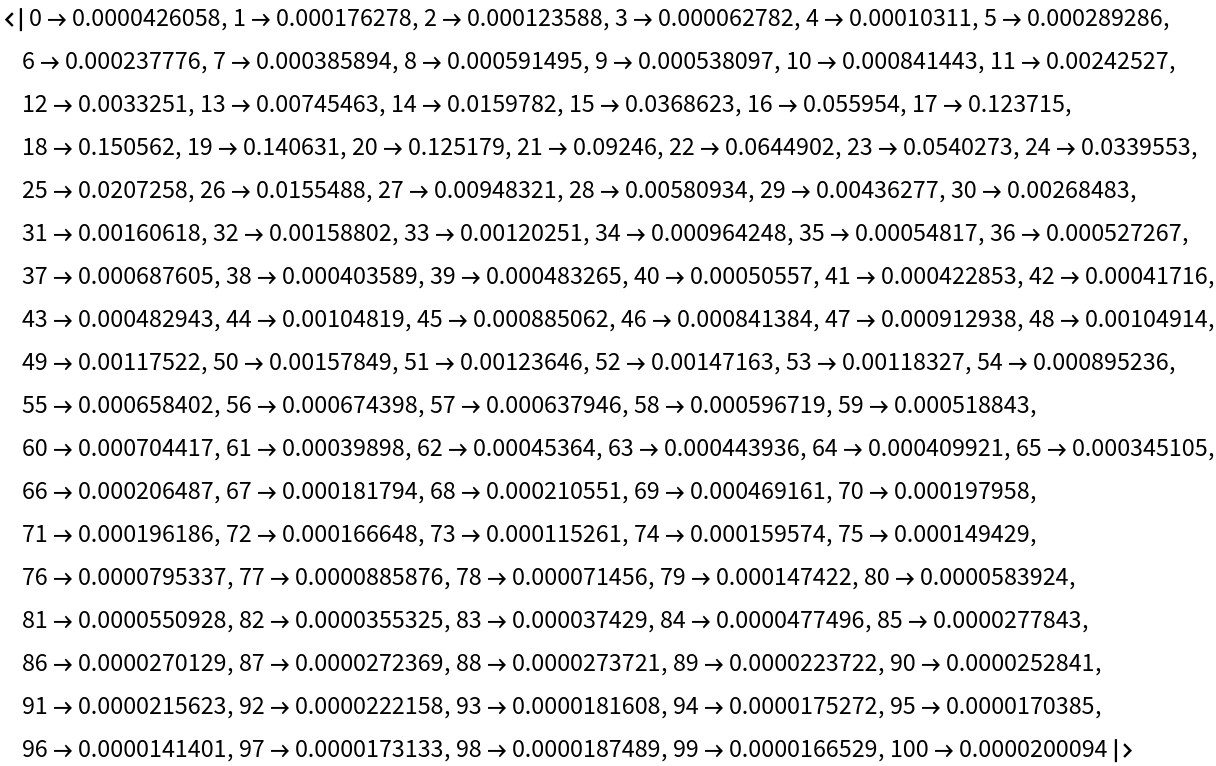Plot the probability distribution over possible ages:

 In:=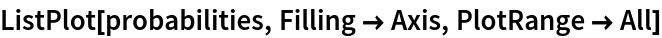Out=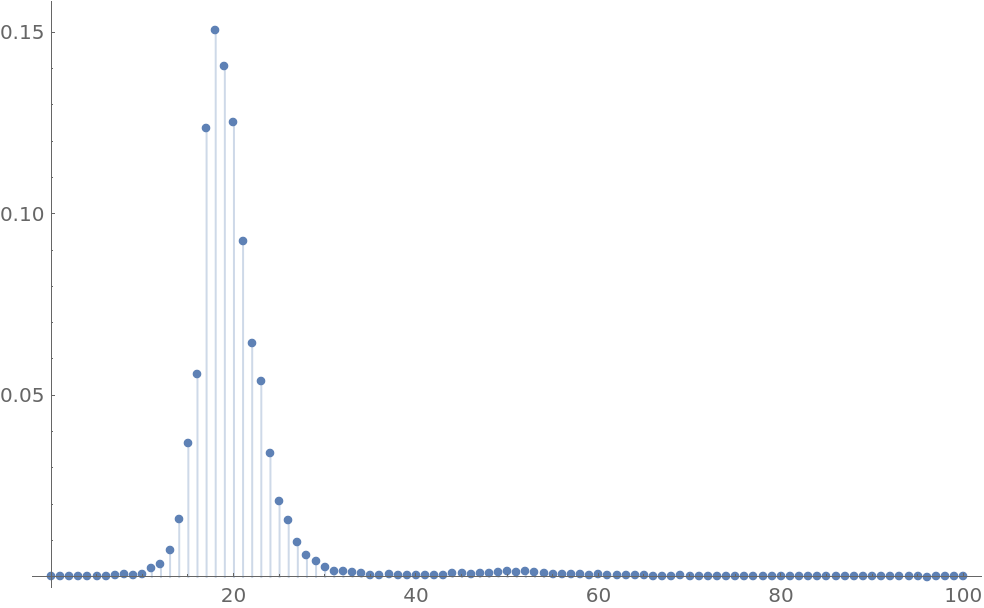The recommended estimator of the age is the mean of the probability mass function:

 In:=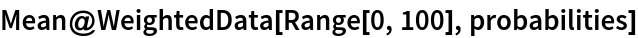Out=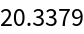By default, the mode is used as the estimator:

 In:=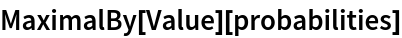Out=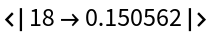This net is designed to work with cropped images of faces only. If the photograph is not a facial image, the results may be unexpected:

 In:=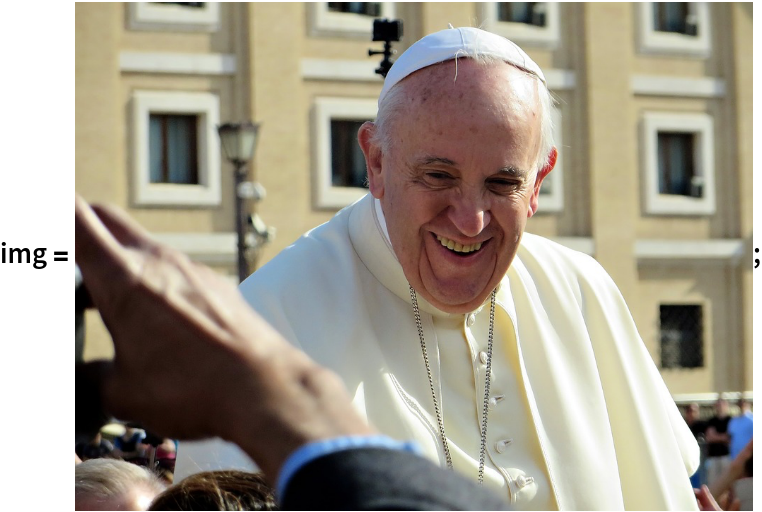In:=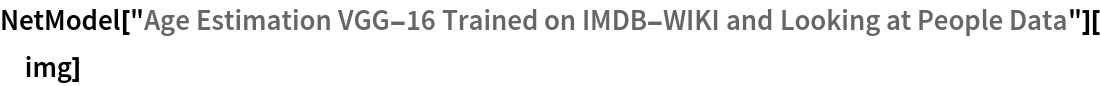Out=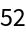Crop the photograph:

 In:=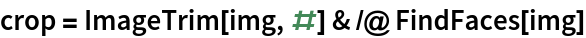Out=Guess the age of a person from the cropped image:

 In:=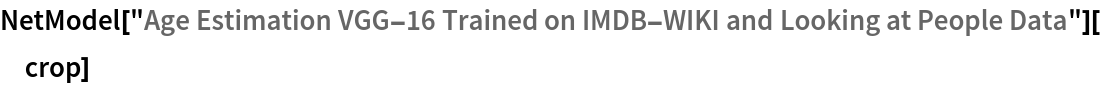Out=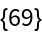### Net information

Inspect the number of parameters of all arrays in the net:

 In:=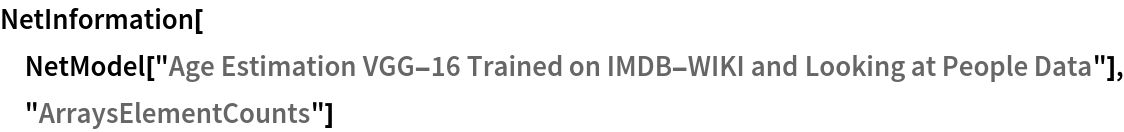Out=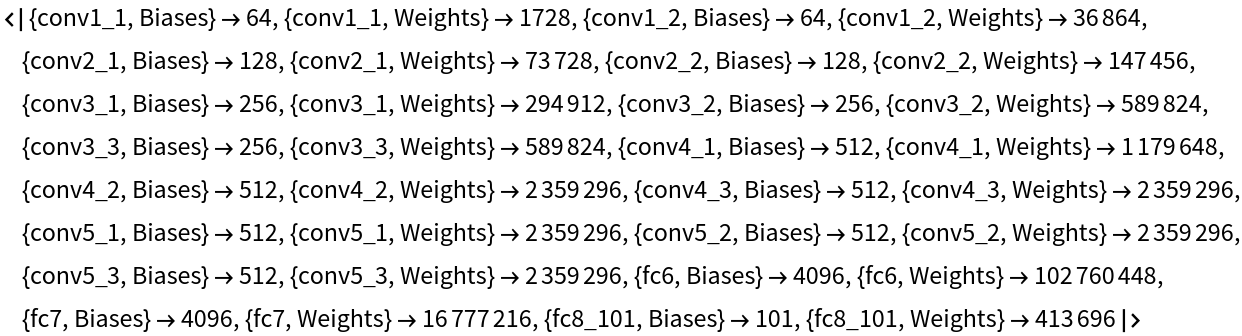Obtain the total number of parameters:

 In:=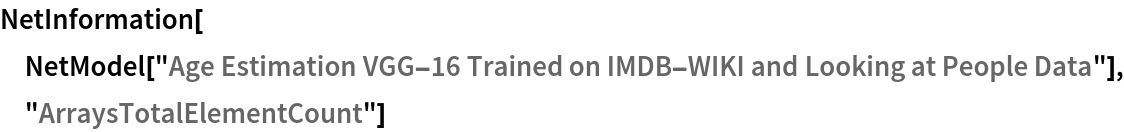Out=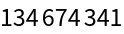Obtain the layer type counts:

 In:=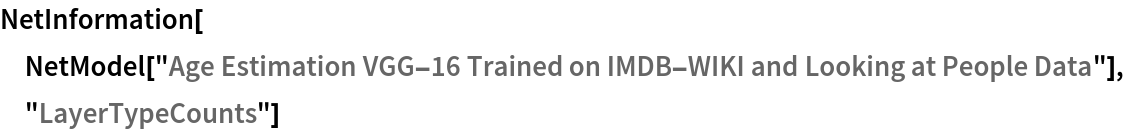Out=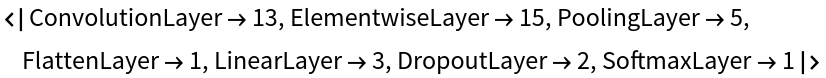Display the summary graphic:

 In:=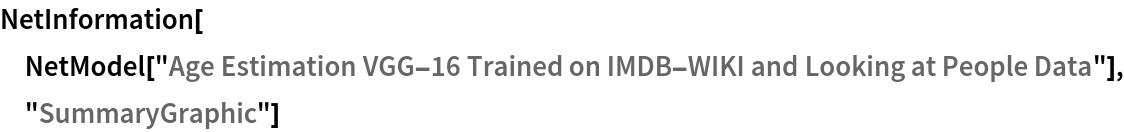Out=### Export to MXNet

Export the net into a format that can be opened in MXNet:

 In:=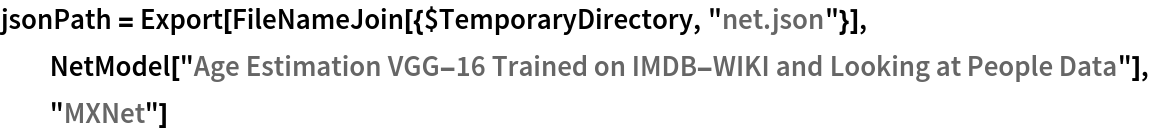Out=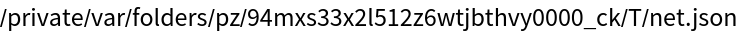Export also creates a net.params file containing parameters:

 In:=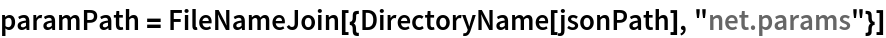Out=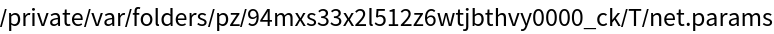Get the size of the parameter file:

 In:=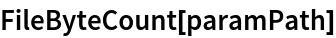Out=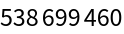The size is similar to the byte count of the resource object:

 In:=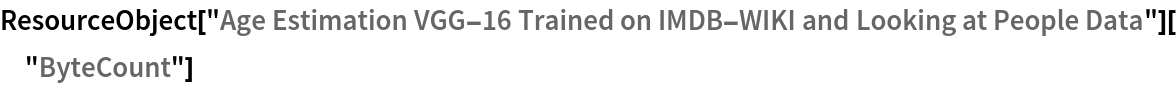Out=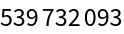Represent the MXNet net as a graph:

 In:=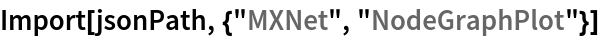Out=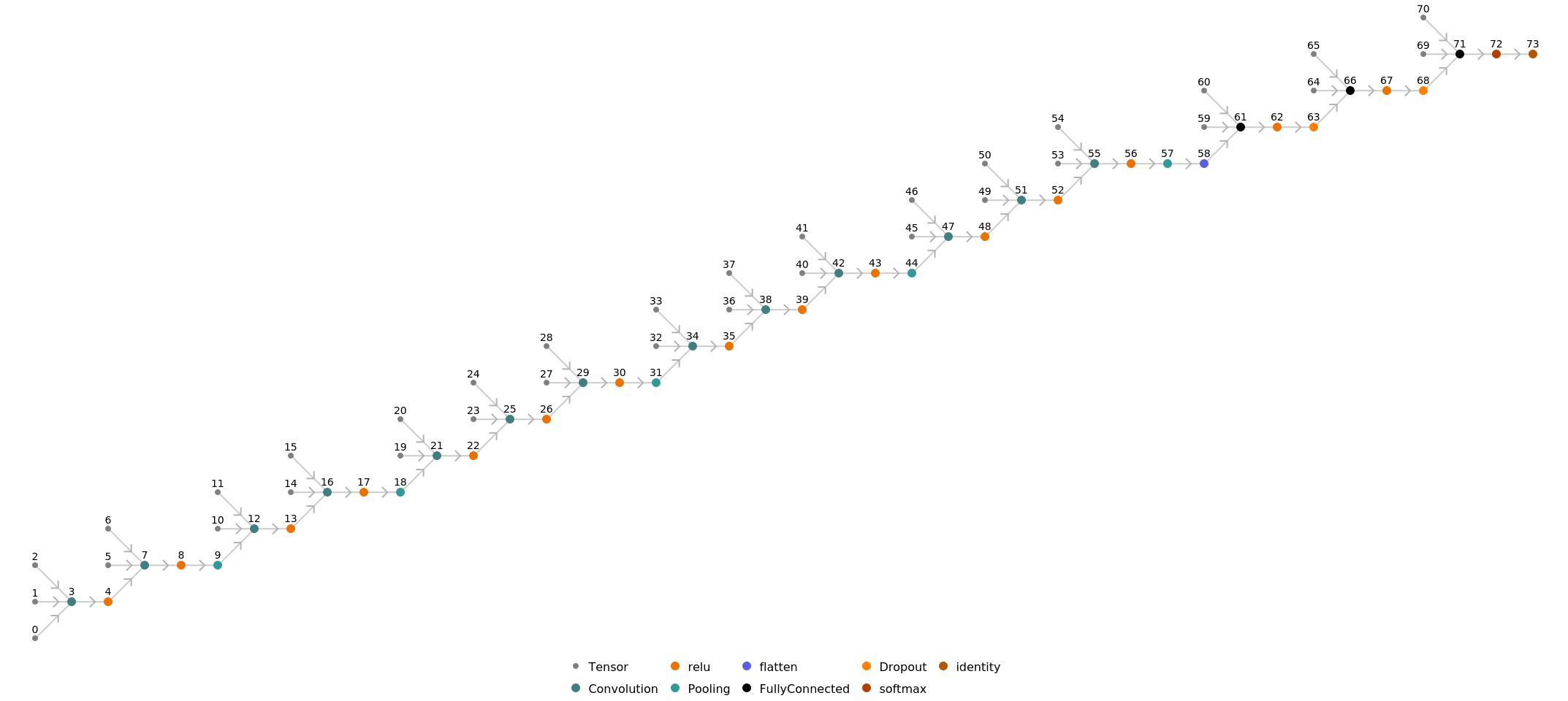## Requirements

Wolfram Language 11.2 (September 2017) or above